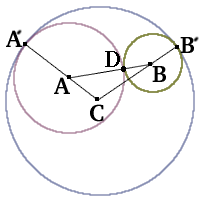#### You may also like### Calendar Capers

Choose any three by three square of dates on a calendar page...Make a set of numbers that use all the digits from 1 to 9, once and once only. Add them up. The result is divisible by 9. Add each of the digits in the new number. What is their sum? Now try some other possibilities for yourself!### Summing Consecutive Numbers

15 = 7 + 8 and 10 = 1 + 2 + 3 + 4. Can you say which numbers can be expressed as the sum of two or more consecutive integers?

# Coins on a Plate

##### Age 11 to 14Challenge Level

Here we use excerpts from several different proofs.We start with the solution fromMolly and Catherine of Mount School, York .

Points C, A and A' are co-linear. To explain this, consider the common tangent to both circles at the point of contact. It is both perpendicular to CA' and to AA' so, as there is a common point in A' , the initial statement is demonstrated.

Molly and Catherine's method proves, in the same way, that C, B and B' are co-linear and A, D and B are co-linear. They finished the proof correctly as did Sarah and Caroline of Ipswich High School and James of Hethersett School, Norfolk. For the following method you may visualise swinging AD round to AA' and BD round to BB'.

Next is the solution byCharlotte and Frances of Ipswich High School

It can be proved that the triangle ABC has perimeter equal to the diameter of the circle centre C because AD = AA' making CAD = CAA' and BD = BB'

so CBD = CBB' . This proves that the perimeter of the triangle ADBCA equals the diameter of the circle because CAA' and CBB' are both radii.

Clare of Maidstone Girls' Grammar School used much the same method as Charlotte and Frances.